## Which of the following statements is NOT true? A. All whole numbers are integers. B. All natural numbers are whole numbers.

Question

Which of the following statements is NOT true?
A. All whole numbers are integers.
B. All natural numbers are whole numbers.
C. All integers are whole numbers.
D All fractions of form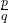, where p and q are integers and q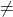0, are rational numbers.

in progress 0
6 months 2021-07-26T13:17:11+00:00 2 Answers 21 views 0

## Answers ( )

1.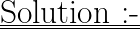⁺˚*･༓☾✧.* ☽༓･*˚⁺‧

╭⋟───────────────

A. All whole numbers are integers.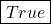➺ Whole numbers include numbers from 0 to above. Integers include all numbers (negative & positive ones). Hence, all whole numbers are integers.

____________________

B. All natural numbers are whole numbers.➺ As said above, whole numbers include numbers from 0 to above. Natural numbers on the other hand include numbers from +1 to above (right side of the number line). So, all natural numbers come under whole numbers.

____________________

C. All integers are whole numbers.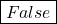➺ Integers include both negative & positive numbers while whole numbers only include numbers from 0 to above (right side of the number line). Since, negative numbers don’t come under whole numbers, integers can’t be categorised as whole numbers. •°• All integers are not whole numbers…..but all whole numbers are integers.

____________________

D. All fractions of form, where p and q are integers and q0, are rational numbers.➺ This is the definition of rational numbers. All fractions are under rational numbers, so this definition stands true for fractions as well.

____________________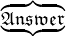⟹ So, the statement which doesn’t match or is NOT true will be ➳ C. All integers are whole numbers.

⁺˚*･༓☾✧.* ☽༓･*˚⁺‧

───────────────⋞╯

ʰᵒᵖᵉ ⁱᵗ ʰᵉˡᵖˢ

꧁❣ ʀᴀɪɴʙᴏᴡˢᵃˡᵗ2²2² ࿐

### C)All integers are whole numbers.

Step-by-step explanation:

### A)

whole numbers are the numbers 0 to +∞ (not including fraction, numbers i.e 6.89 etc)

integers are the numbers -∞ to +∞ (not including fraction, decimal numbers i.e -5.57, 7.74 etc)

since integers are the numbers including whole numbers thus the statement is true

### B)

Natural numbers are the numbers 1 to +∞ (not including fraction, decimal numbers i.e 6.89 etc)whereas whole numbers are the numbers 0 to +∞ (not including fraction, numbers i.e 6.89 etc)

hence, the statement is true

### C)

All Whole numbers are integers but not all integers are whole numbers

therefore, the statement is not true

### D)

the given definition is the perfect definition of rational numbers

hence, the statement is true